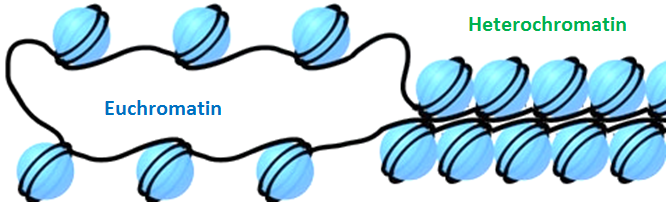# Random walk, brownian motion, and diffusion¶

This notebook illustrates some interesting applications of random walk models in biology

## I. Polymer models of noncondensed chromosomes¶For this toy model of chromosomes (the Rouse model), import a pre-existing python package "rouse"

The basic logic of the Rouse model is very simple. Each monomer wiggles in space due to thermal fluctuation ("random walk" dynamics). In addition to this, each pair of bonded monomers attract each other like a spring with a vanishingly small relaxation length ("non-random" dynamics)Two parameters to initialize the model: $N$ is the number of monomers, and $d$ is space dimension (default=3)

Here we show some equilibrium conformations of the polymer chain under the Rouse model

The equilibrium conformation of the Rouse model is a collapsed polymer. Let's inspect the collapse process when we evolve a stretched chain freely

Despite the collapse, neighboring monomers are more likely to "contact each other". That's because they are more tightly linked and so their spatial positions are more correlated. In modern chromosomal research, this information is captured by the so-called "HiC" analysis). A standard output is the following contact probability map between each pair of loci on a DNA segment.